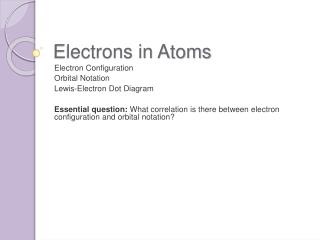DownloadDownload PresentationElectrons in Atoms

# Electrons in Atoms

Télécharger la présentation## Electrons in Atoms

- - - - - - - - - - - - - - - - - - - - - - - - - - - E N D - - - - - - - - - - - - - - - - - - - - - - - - - - -
##### Presentation Transcript

1. Electrons in Atoms Electron Configuration Orbital Notation Lewis-Electron Dot Diagram Essential question: What correlation is there between electron configuration and orbital notation?

2. Essential question: What correlation is there between electron configuration and orbital notation? Electron Configuration • A form of notation which shows how the electrons are distributed among the various atomic orbital and energy levels • Example of e- configuration for Hydrogen: • 1s1 Total # of electrons in that sublevel. 1st column of s-block S-Block Principle Quantum number which stands for the energy level

3. Periodic Patterns • Yellow represents the s-block • Pink represents the p-block • Green represents the d-block • Orange represents the f-block S-block S-block d-block p-block f-block

4. Learning Check • What element would have an electron configuration ending in 4s1? • Potassium

5. Order of filling sublevels • 1s2 2s2 2p6 3s2 3p6 4s2 • You must fill in each sublevel with its maximum number of electrons before you move to the next energy level

6. Examples • e- configuration for Oxygen • Oxygen has 8 electrons and is in period 2 • 1s2 2s2 2p4 6 valence electrons 2 energy level and located in the p-block

7. Practice • Write the e- configuration for the following elements: • Helium • Neon • Sulfur • Calcium

8. Orbital Notation • Every box represents an orbital • Every arrow represents electrons

9. General Rules • Pauli Exclusion principle • Every orbital can only hold 2 electrons with opposite spins • Hund’s rule • Within a sublevel, place one e- per orbital before pairing them. Right WRONG

10. Example: 1s2 2s2 2p4 • Orbital Notation for Oxygen: • You know the e- configuration for oxygen is 1s22s22p4 • Remember: • Every orbital is represented by a box • Every orbital holds a maximum of 2 electrons • s-block represented by 1 orbital • p-block represented by 3 orbitals

11. Practice • Show orbital notation for the following elements: • Helium • Neon • Sulfur • Calcium

12. Lewis Electron Dot Structure • An atom’s Lewis electron dot structure consists of : • element’s symbol-which represents the atomic nucleus and inner-level electrons, surrounded by dotsrepresentingthe atom’s valence electrons. Source: Glencoe Chemistry Matter and Change pg. 140

13. Group 1A: 1 valence electron Group 3A: 3 valence electrons Group 4A: 4 valence electrons Group 5A: 5 valence electrons Group 6A: 6 valence electrons Group 7A: 7 valence electrons Group 8A: 8 valence electrons Group 2A: 2 valence electrons How do we use the periodic table to draw Lewis electron dot structures? Identifying the representative group number will allow you to determine the number of valence electrons. Here’s how….

14. Writing out Lewis Electron Dot Structure • Group # = number of valence electrons • Example: Phosphorus is on group 5A, so it has 5 valence electrons. Step 3: Continue placing one electron on each of the four sides of the symbol & then pair up the electrons until you have placed all valence electrons around the symbol Step 1: write the element symbol P Step 2:Start at the top of the symbol and place one electron

15. Practice • Write the Lewis Electron Dot Structure for the following elements: • Helium • Neon • Sulfur • Calcium

16. Questions??